Russia, St.-Petersburg, Department of Mathematics, St.-Petersburg State Technical University

E-mail: noghin@osipenko.stu.neva.ru

RELATIVE IMPORTANCE OF CRITERIA IN A FUZZY CASE

Abstract. Elements of quantitative theory of relative importance of criteria in case of fuzzy preference relation are presented. The basic mathematical definition of relative importance of criteria generalizes one, introduced by the author in nonfuzzy case. The main result of the pa- per contains an upper estimate for unknown fuzzy set of non-dominated vectors.

Владимир Д. Ногин

Россия, Санкт-Петербург, кафедра математики СПбГТУ Эл. почта: noghin@osipenko.stu.neva.ru

ОТНОСИТЕЛЬНАЯ ВАЖНОСТЬ КРИТЕРИЕВ В НЕЧЕТКОМ СЛУЧАЕ

Аннотация. Представлены элементы теории относительной важности критериев в случае нечеткого отношения предпочтения. Основное математическое определение относительной важности обобщает определение, введенное автором в четком случае. Главный результат работы содержит оценку сверху для неизвестного нечеткого множества недоминируемых векторов.

1. INTRODUCTION. Usually a decision-maker (DM) possesses certain information on relative importance of criteria. A lot of authors characterize the importance of criteria by means of special positive numbers called importance coefficients. These authors do not try to give any rigorous definitions for the coefficients. As a rule, each author proposes his own procedure to obtain the coefficients and different procedures lead to distinct results. They are interested in obtaining the coefficients but they do not analyze the nature of the relative importance.

What do we mean by "criterion i is more important than criterion] "? How may we use a numerical information on relative importance of criteria when a fuzzy preference relation of the DM is unknown?

This paper being an extension of deals with problems relating to above questions. We shall give a formal definition of the concept of relative importance in a fuzzy case and formulate new original results to construct an upper estimate for a fuzzy set of non-dominated vectors. Some examples illustrate the theoretical results.

2. NOTATION AND PRELIMINARY DEFINITIONS. Let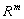be an т –dimensional vector space,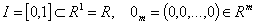.

Recall that a map m :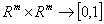is called a (binary) fuzzy relation. A fuzzy relation m (· ,· ) are said to be

irreflexive, if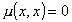asymmetric, if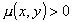implies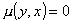transitive, if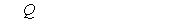• invariant with respect to positive linear transformation, if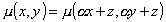for any.Let m be a fuzzy relation. The relation m is called a (fuzzy) cone relation, if there exists a fuzzy cone l :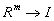(i.e.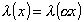for all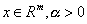), such that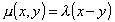for any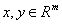.

We shall use superscripts to indicate the vector and subscripts to indicate the components of such vector.

3. BASIC DEFINITIONS. Consider a mathematical model of choice consisting of the three objects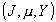, where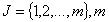is a number of criteria, m :is an asymmetric fuzzy relation of the DM,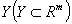is a set of feasible vectors.

If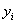is preferred to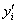with respect to the i-th criterion (i.e.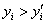), then the positive numberindicates a "degree" of the preference.

Further we shall suppose the fuzzy relation m satisfies Pareto axiom, i.e. for all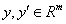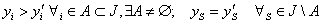imply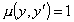.

Definition 1. Let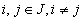. A criterion i we shall call more important than a criterion j with two given positive parameters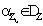and a grade of confidence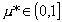, if for all vectorsrelations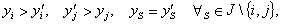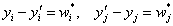imply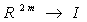. The number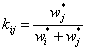is called a coefficient of relative importance.

Example. Let т =3. Assume that the first criterion is more important than the second one with the coefficient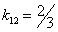and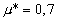. Then, for instance, the vector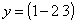is more preferred thanand also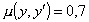, because of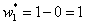,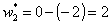and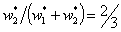.

4. USE OF INFORMATION ON RELATIVE IMPORTANCE. As above let Y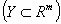be a set of feasible vectors and m :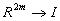be an asymmetric fuzzy relation (which as a rule in practice is unknown). The fuzzy set whose a membership function is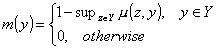is called a fuzzy set of non-dominated vectors . This notion is an extension of well-known concept of (non fuzzy) set of non-dominated vectors .

Theorem 1. Let p. be an irreflexive transitive and invariant fuzzy relation, which satisfies Pareto axiom. Suppose that a criterion i is more important than a criterion j with a coefficient of relative importance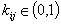and a grade of confidence. Then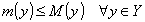(1)

where M is a membership function of a fuzzy set of non-dominated vectors with respect to the fuzzy relation l :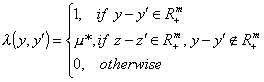Here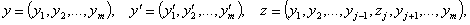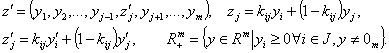The inequality (1) shows that a fuzzy set with the membership function т is a subset of the fuzzy set with the membership function M, i.e. the second set is an upper estimate for the first one. Thus, when the fuzzy preference relation p, is unknown, due to Theorem 1 we may construct an upper estimate M(y) for a fuzzy set of non-dominated vectors m(y) using in- formation on relative importance of criteria.

5. EXAMPLE. Let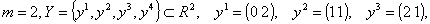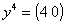. Evidently, in this case a Pareto set is, since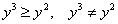.

Suppose that we have got an additional information on relative importance of criteria. Namely, the first criterion is more important than the second one with the coefficient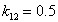and the grade of confidence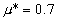. Then in according to Theorem 1 we have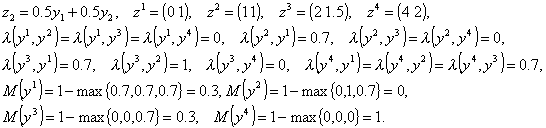Hence, in this case the fuzzy set of non-dominated vectors is given as follows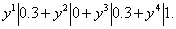It is an upper estimate for the unknown fuzzy set of non-dominated vectors with respect to preference relation m . It is easy to see that the vector y4 is the most preferable for the DM in according to the additional information on relative importance of criteria.

6. GENERAL CASE. In a general case let us introduce the following definition.

Definition 2. A group of criteria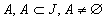we shall call more important than a group of criteria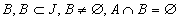, with given positive parameters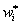(for all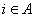),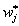(for all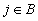) and a grade of confidence, if for all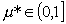relations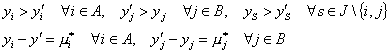imply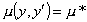.

As above the number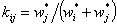we shall call a coefficient of relative importance for alland.

It can be proved the following result.

Theorem 2. Let m be an irreflexive transitive and invariant fuzzy relation, which satisfies Pareto axiom. Suppose that a group of criteria A is more important than a group of criteria В , with the coefficients of relative importance for all,and a grade of confidence. Then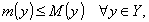where M is a membership function of a fuzzy set of non-dominated vectors with respect to the fuzzy relation l :with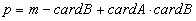and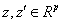. Here the first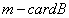components of the vector z are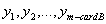and tne otner components are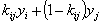for all,(similarly for the vector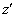).

Corollary. By hypotheses of Theorem 2 the maximal value of p is attained in the case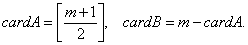Here the brackets [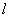] denote an integral part of number.

For example, if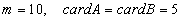, then p = 10 - 5 + 25 = 30. Thus, in order to construct an upper estimate for a fuzzy set of non-dominated vectors, when a group consisting of five criteria is more important than other group consisting of five criteria, in according to Theorem 2 we have to deal with a mathematical model which contains thirty criteria and also twenty five among of them are new.

REFERENCES

 Noghin, V.D. (1997), Relative importance of criteria: a quantitative approach. Journal of Multi-Criteria Decision Analysis, 6, pp. 355-363.

 Orlovsky, S.A. (1978), Decision making with a fuzzy preference relation. Fuzzy Sets and Systems, 1,pp. 155-167.

 Yu,P.L. (1985), Multiple-Criteria Decision-Making: Concepts, Techniques, and Exten- sions, New-York: Plenum.

 Noghin, V.D. (1994), Upper estimate for a fuzzy set of nondominated solutions. Fuzzy Sets and Systems, 67, pp. 303-315.

 Site of Information Technologies Designed by  inftech@webservis.ru.